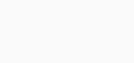# Best turns ratio meterThe transformer turns ratio is the number of turns of the primary winding divided by the number of turns of the secondary winding.

The transformer turns ratio provides the expected operation of the transformer and the corresponding voltage required on the secondary winding.The voltage ratio of an ideal transformer is directly related to the turns ratio:The current ratio of an ideal transformer is inversely related to the turns ratio:This is very basic formula to calculate the Transformer Turns Ratio.

Where ,

Vp = primary voltage, Ip = primary current,

Vs = secondary voltage, Is = secondary current,

Np = number of turns in the primary winding and

Ns = number of turns in the secondary winding.

This is very basic method of testing transformer turns ratio of a single phase winding.

If we apply the Voltage form the Primary winding of the Transformer and measure back through voltage from Secondary side then by measuring the Ratio between Input & Output voltage is the Turns Ratio of that winding/phase.

You can see that the Voltage is directly proportional to the Number of Turns of Windings.

So we can say that,

In the step up Transformer the Number of Turns at Secondary side is more than the number of Primary side.

Where in the step down transformer the Number of Turns at Secondary side is less than the number of Primary side.

Types of Turns Ratio Tests.

TR (turns ratio)

This test energizes any chosen winding at a specified voltage and measures the induced voltage on any other winding.The results are then presented as a ratio (e.g. 3:1, 4:1, etc.) ,

TRL (turns ratio by inductance)

This test separately energizes two selected windings and measures the inductance value of each winding.
The results are then presented as a ratio of turns (e.g. 3:1, 4:1, 5:1, etc.) calculated from the squared root of the inductance values.
Phase is also measured: ‘in- phase’ (positive polarity) and ‘anti-phase’ (negative polarity).

LVOC (low voltage open circuit)

This test applies a voltage to the primary winding, reads the voltage induced in the secondary winding and presents the results as a secondary voltage (e.g. 2.35 V).
Phase is also measured: ‘in- phase’ (positive polarity) and ‘anti-phase’ (negative polarity).

VOC (voltage open circuit only)

This test uses the same principal as LVOC but by using a high-power generator, capable of energizing a winding at voltages up to 270V.
The test is suitable for testing low-frequency power transformers.
Phase is also measured: ‘in- phase’ (positive polarity) and ‘anti-phase’ (negative polarity).

VOCX (voltage open circuit with external source only)

This test, which is used in conjunction with the Voltech AC Interface Fixture.
This will control an external AC source or step-up transformer for testing higher power and higher voltage transformers up to 600V and 10A.
Phase is also measured: ‘in-phase’ (positive polarity) and ‘anti-phase’ (negative polarity).

For 3 Phase Turns Ratio Measurement The Vector Group is Very Important factor.

As we are aware, transformer is a device which converts power at one voltage level to power at another voltage level. By the rated voltage ratio, one can know the magnitude of the rated primary and secondary voltages and by the rated frequency rating of the transformer, one can know the rate of change of these voltages. But, it is also important to know the phase relationship between the HV side voltage and the LV side voltage. This phase relationship between the HV side and LV side voltages is denoted by the vector symbol of the transformer.

Winding Connections :

Winding Connections: